# How to Calculate and Solve for Electrode Potential | Nernst Equation | Corrosion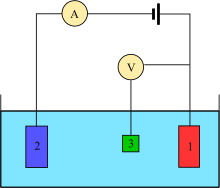The image above represents electrode potential.

To compute for electrode potential, three essential parameters are needed and these parameters are Standard Electrode Potential (Eo), Number of Electrons (n) and Molar Activity (Cion).

The formula for calculating electrode potential:

E = Eo0.059/nIn(Cion)

Where:

E = Electrode Potential | Nernst Equation
Eo = Standard Electrode Potential
n = Number of Electrons
Cion = Molar Activity

Let’s solve an example;
Find the electrode potential when the standard electrode potential is 14, the number of electrons is 10 and the molar activity is 12.

This implies that;

Eo = Standard Electrode Potential = 14
n = Number of Electrons = 10
Cion = Molar Activity = 12

E = Eo0.059/nIn(Cion)
E = 14 – 0.059/10In(12)
E = 14 – (0.0059)(2.48)
E = 14 – 0.0146
E = 13.98

Therefore, the electrode potential is 13.98 V.

Nickzom Calculator – The Calculator Encyclopedia is capable of calculating the electrode potential.

To get the answer and workings of the electrode potential using the Nickzom Calculator – The Calculator Encyclopedia. First, you need to obtain the app.

You can get this app via any of these means:

To get access to the professional version via web, you need to register and subscribe for NGN 2,000 per annum to have utter access to all functionalities.
You can also try the demo version via https://www.nickzom.org/calculator

Apple (Paid) – https://itunes.apple.com/us/app/nickzom-calculator/id1331162702?mt=8
Once, you have obtained the calculator encyclopedia app, proceed to the Calculator Map, then click on Materials and Metallurgical under Engineering.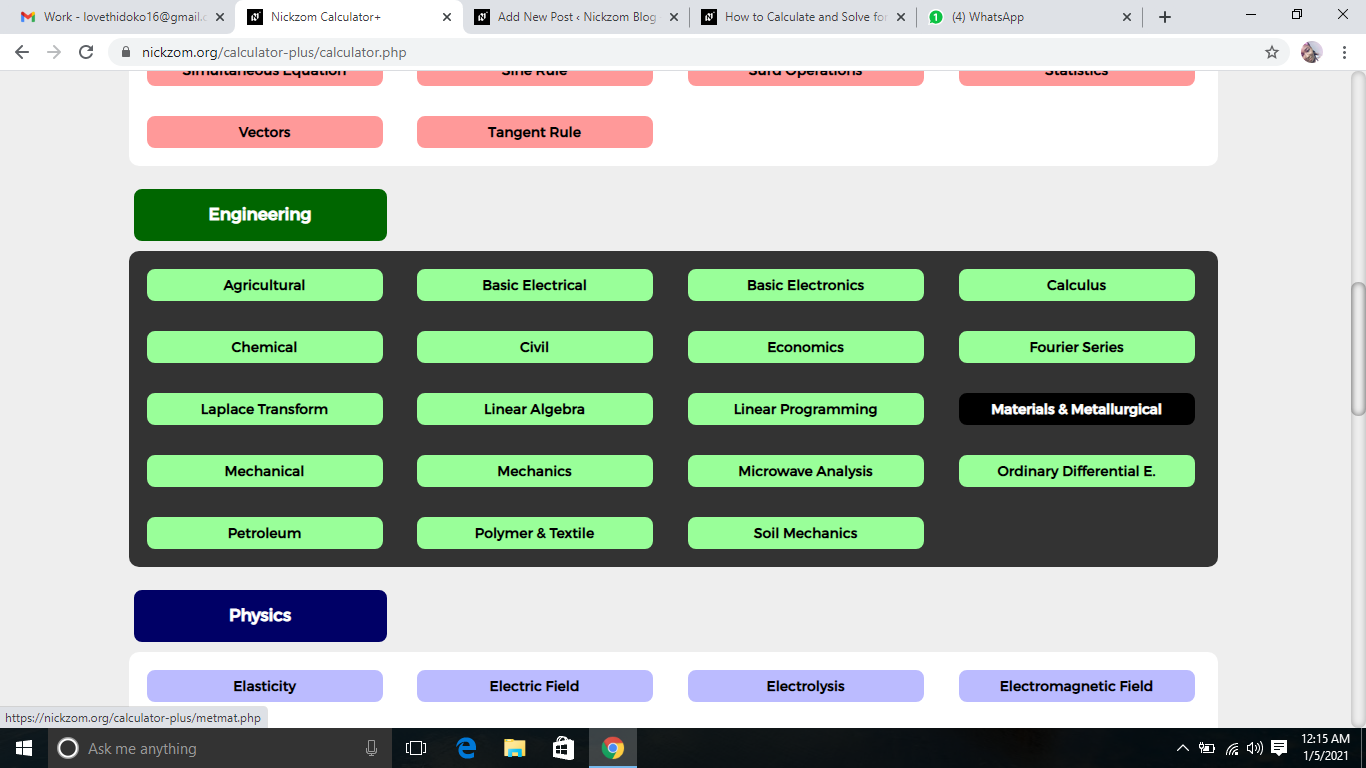Now, Click on Corrosion under Materials and Metallurgical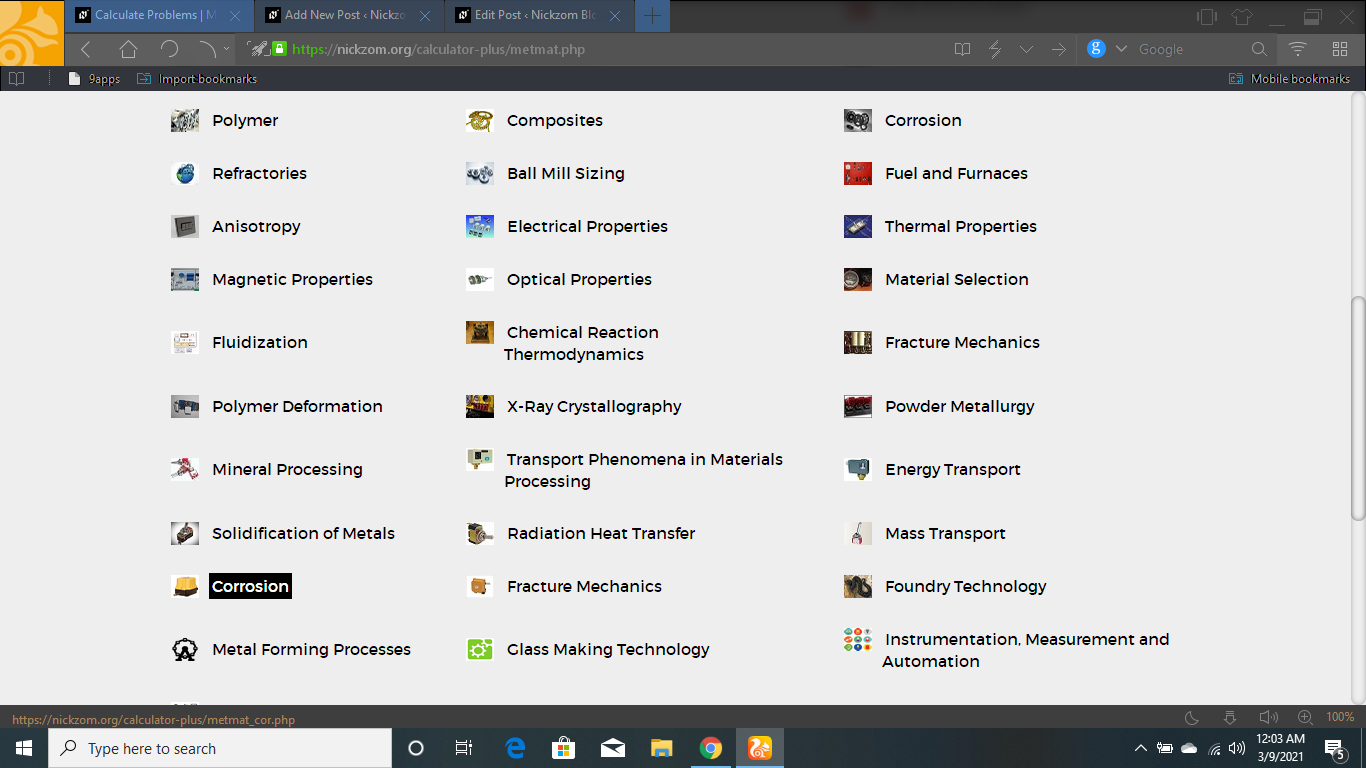Now, Click on Electrode Potential under Corrosion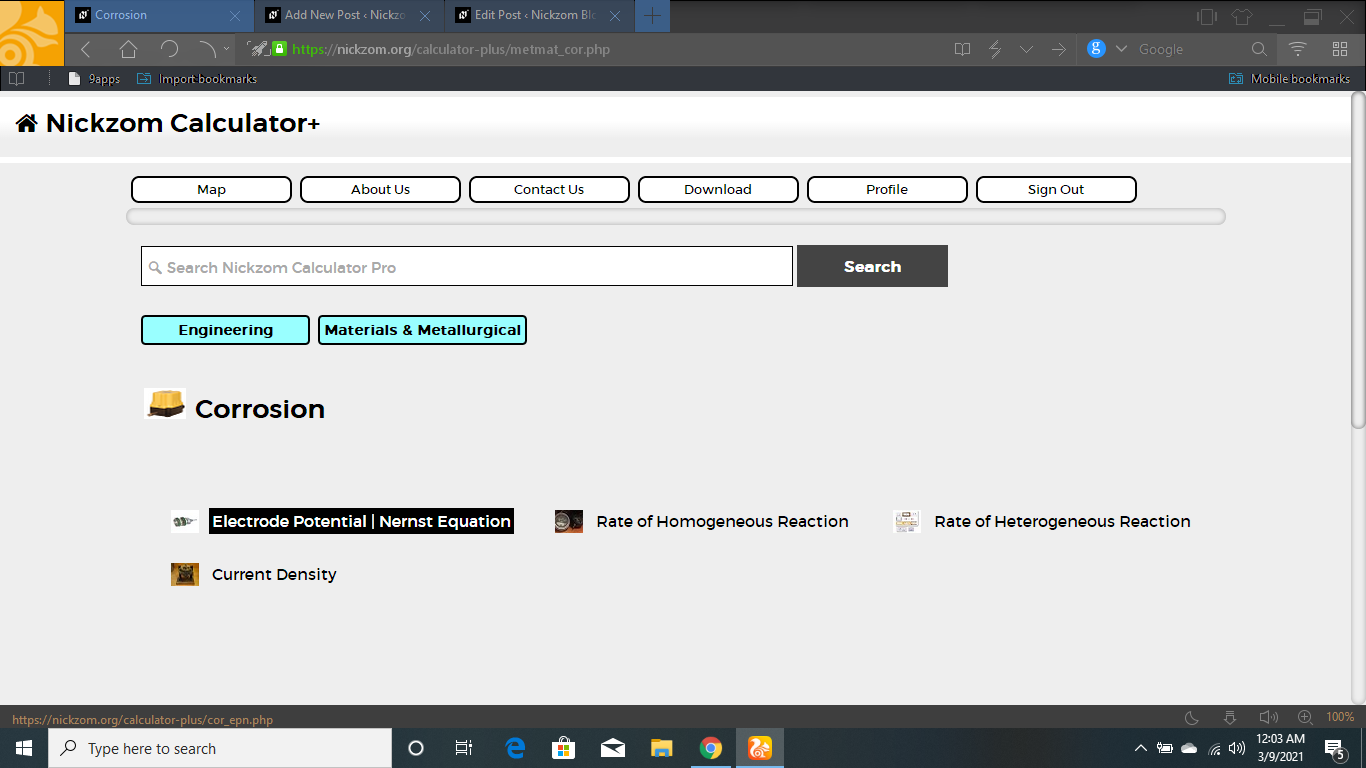The screenshot below displays the page or activity to enter your values, to get the answer for the electrode potential according to the respective parameters which is the Standard Electrode Potential (Eo), Number of Electrons (n) and Molar Activity (Cion).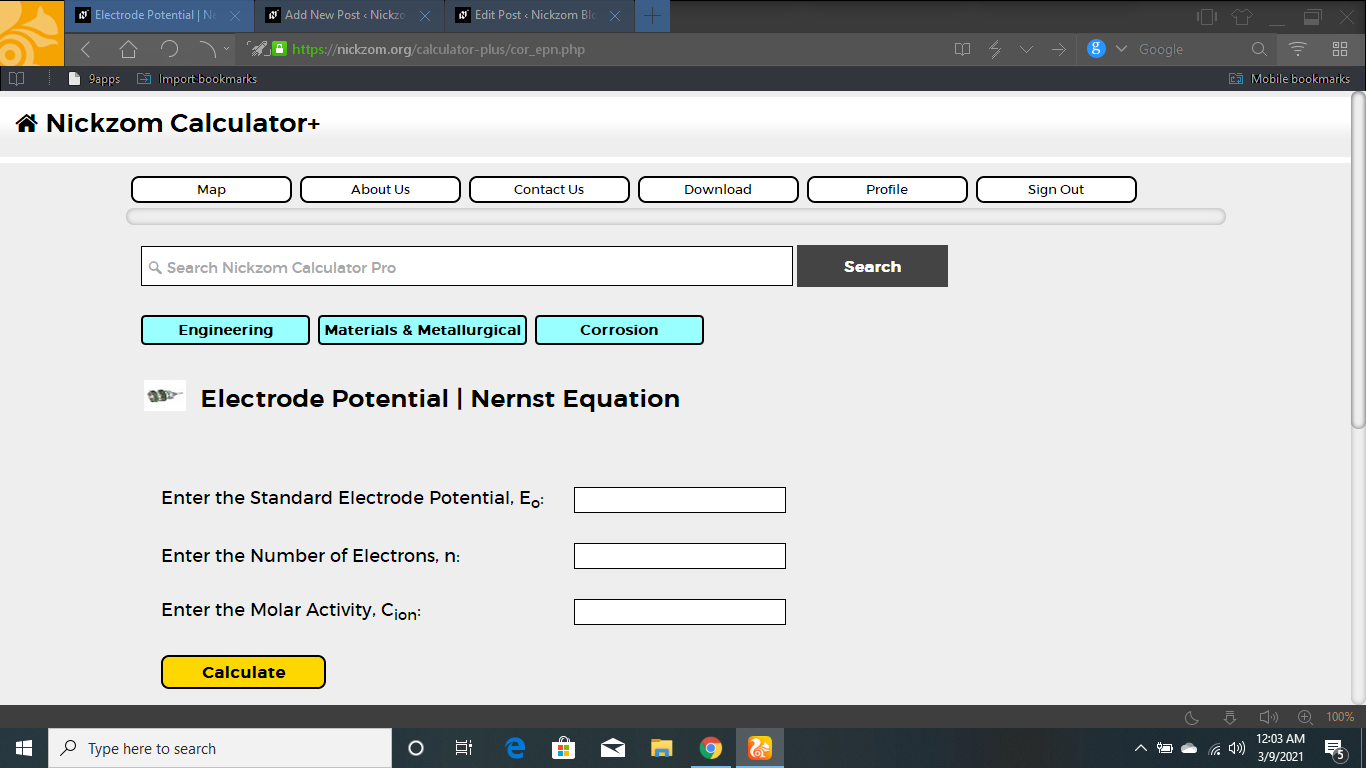Now, enter the value appropriately and accordingly for the parameter as required by the Standard Electrode Potential (Eo) is 14, Number of Electrons (n) is 10 and Molar Activity (Cion) is 12.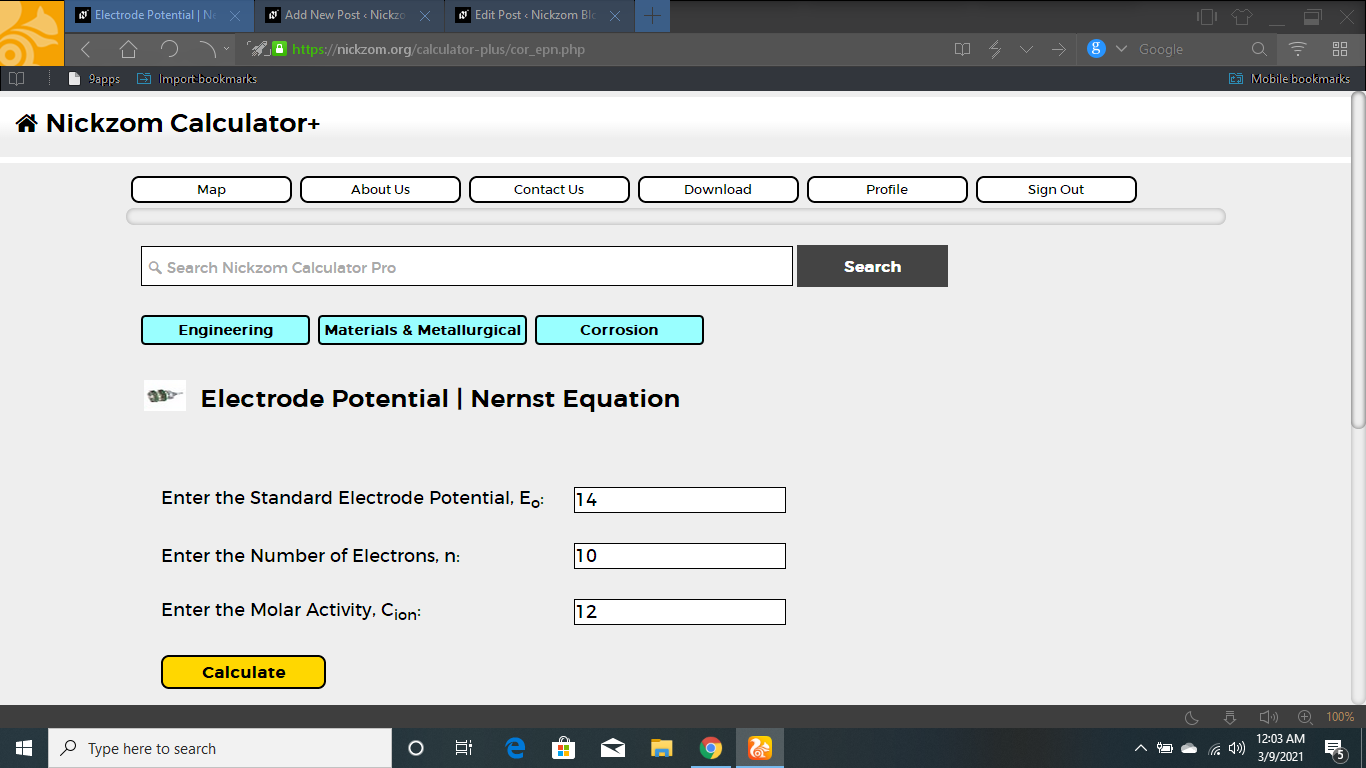Finally, Click on Calculate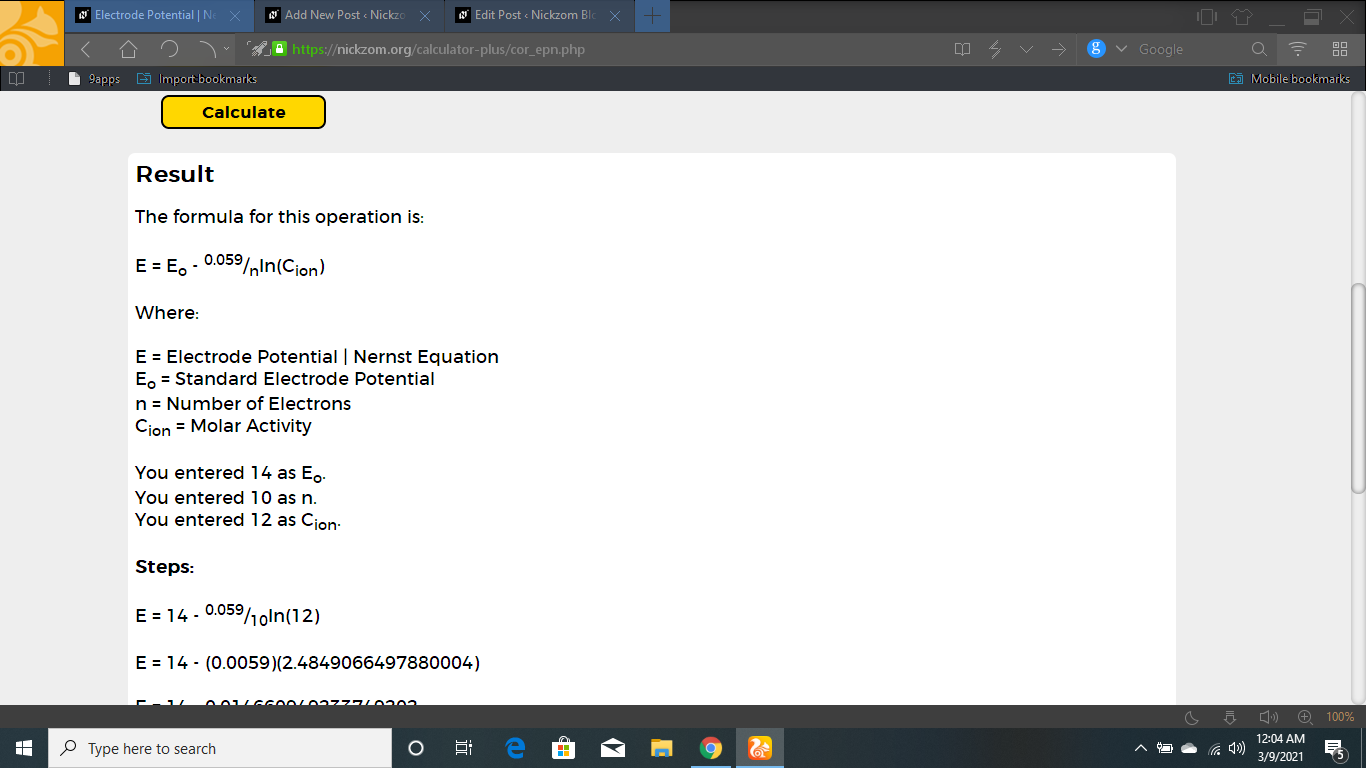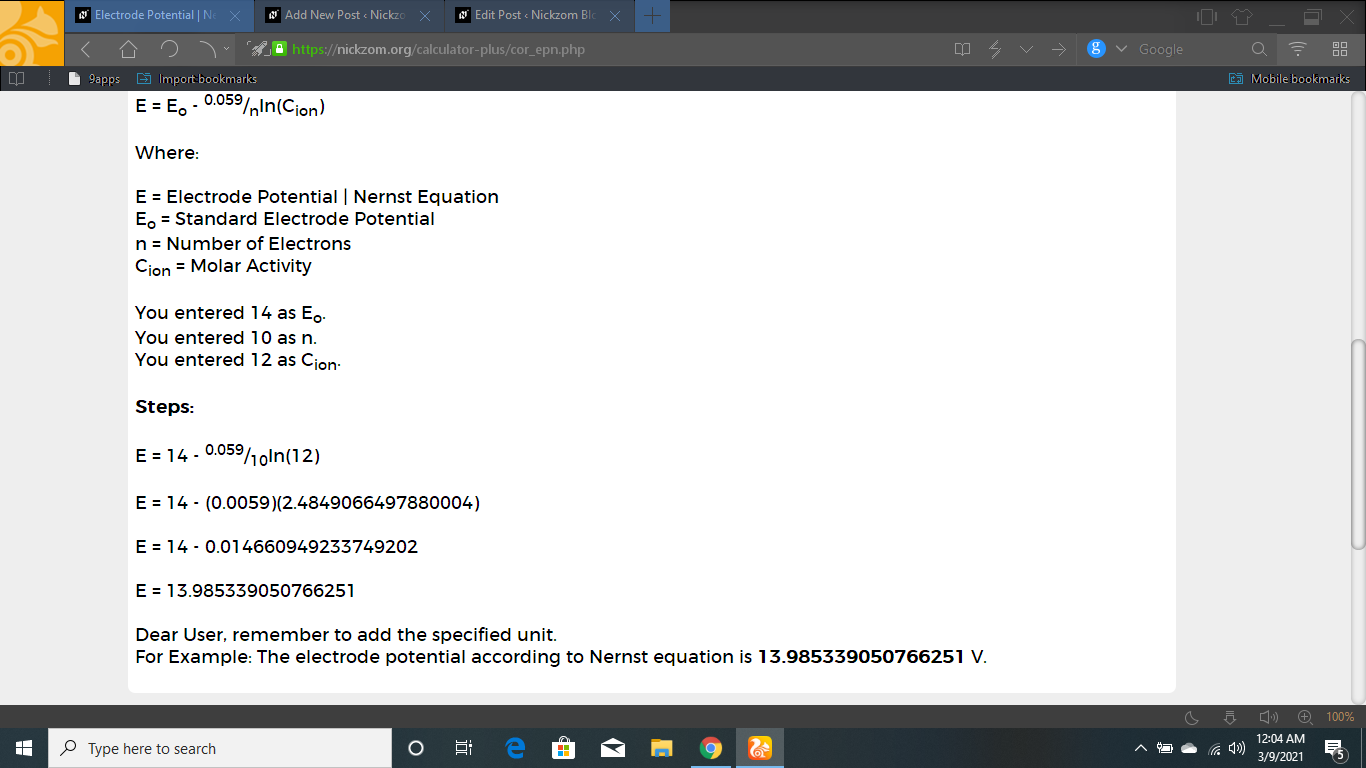As you can see from the screenshot above, Nickzom Calculator– The Calculator Encyclopedia solves for the electrode potential and presents the formula, workings and steps too.